# sScale

sScale is an instrument for real-time visual and aural exploration of harmonically resonant noise. The program is controlled with the mouse and keyboard to change various parameters of the sound processing algorithm, such as pitch, and attributes of the rendered graphics, such as color and blur amount. The underlying source of data is three independent channels of pink noise running through a highly resonant comb filter.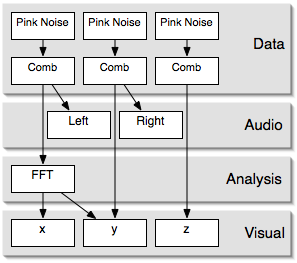sScale Flow Diagram

Pink noise is generated by summing 12 octaves of white (uniform) noise. The octaves of white noise are produced by generating and holding a unform random value every 2n samples, where n=0 is the highest octave. A feedback comb filter is simply a signal summed with a time delayed output of the filter.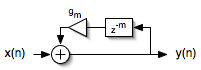Feedback Comb Filter

The feedback in the system causes linearly spaced resonant nodes, harmonics, in the frequency spectrum. Harmonics of some frequency, f, are defined with the equation hn = nf where n is the set of all positive integers. Using positive feedback with a comb filter produces even harmonics and using negative feedback generates odd harmonics. By controlling the delay time of the comb filters, the pitch of the resultant sound can be modified.

The audio samples are represented visually in two different ways. The first of these is a time-domain phase plot which maps the left-channel samples to the x-axis, the right-channel samples to the y-axis, and the third, unheard, channel to the z-axis. In this mode, Lissajous figures resembling clouds of particles and complex tangled strings are produced. Curiously, by making the filter create odd harmonics, the figures display a high degree of spatial symmetry. Using positive feedback, i.e. even harmonics, makes curves that are completely asymmetric.Symmetric Form(odd harmonics)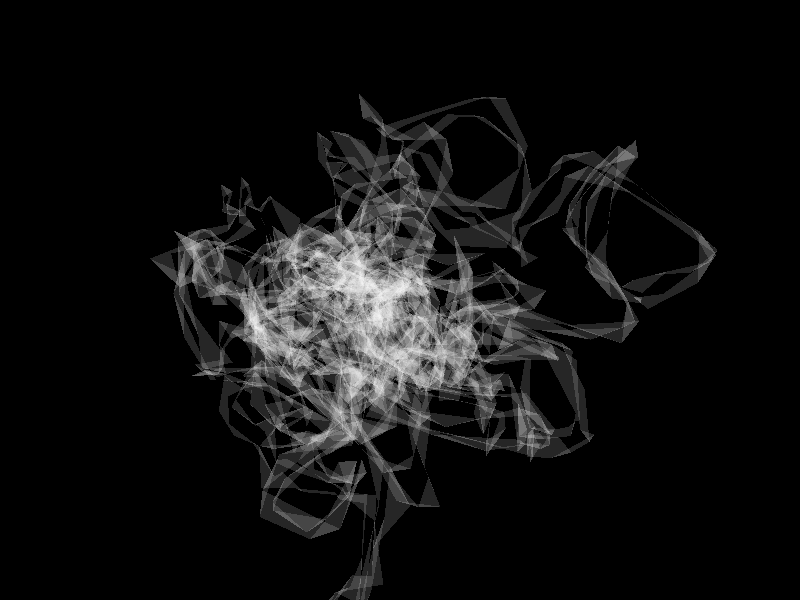Asymmetric Form(even harmonics)

The second mode of visualization is a polar plot of the left-channel data in the frequency domain. An FFT is performed on the time-domain data and the magnitude and phase of the sinusoids is plotted. This mode creates a ring-like cloud of rapidly orbiting particles. By muting the noise going into the comb filter, the particles spiral inward forming something like a galaxy.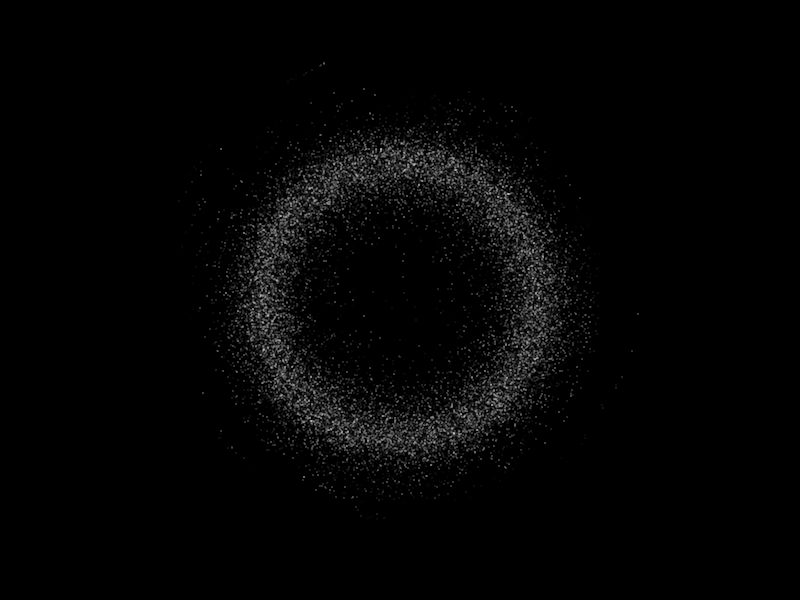Frequency DomainRing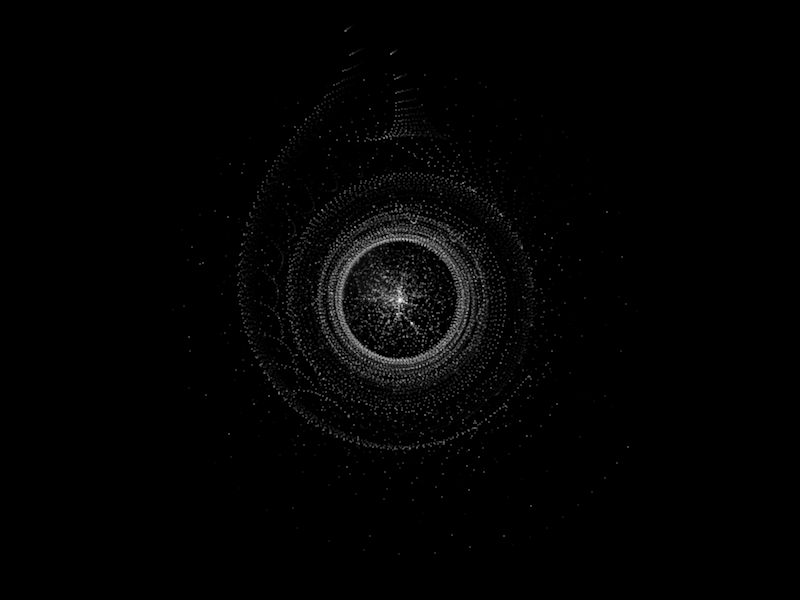Muting Filter Input

Screenshots
TD = time-domain
FD = frequency-domain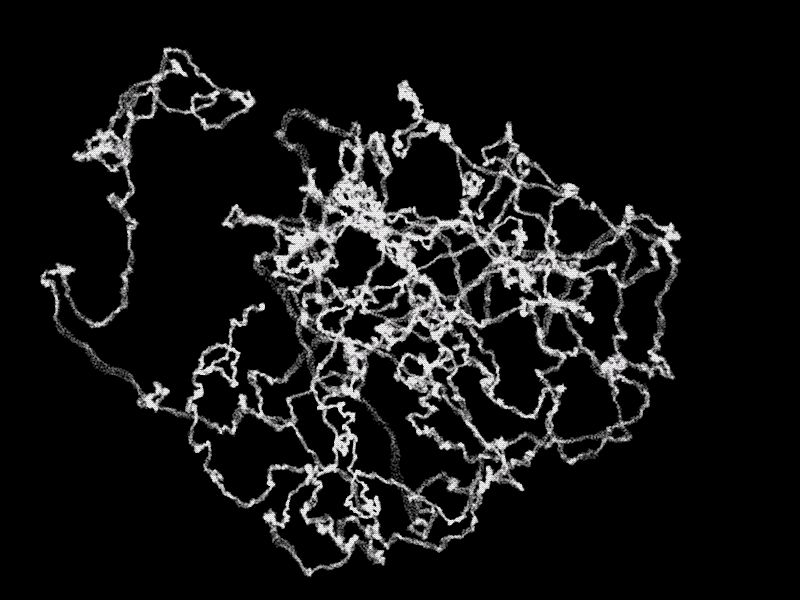TD [Random Walk]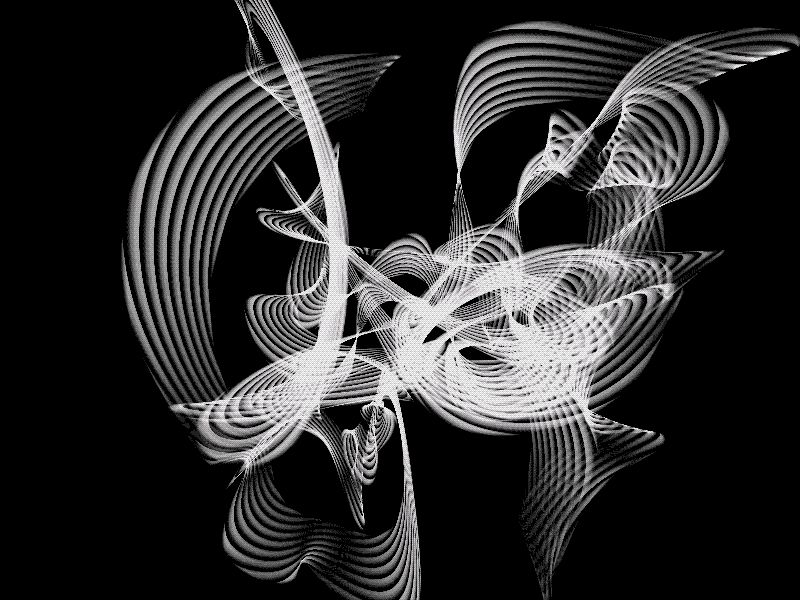TD [Harmonograph]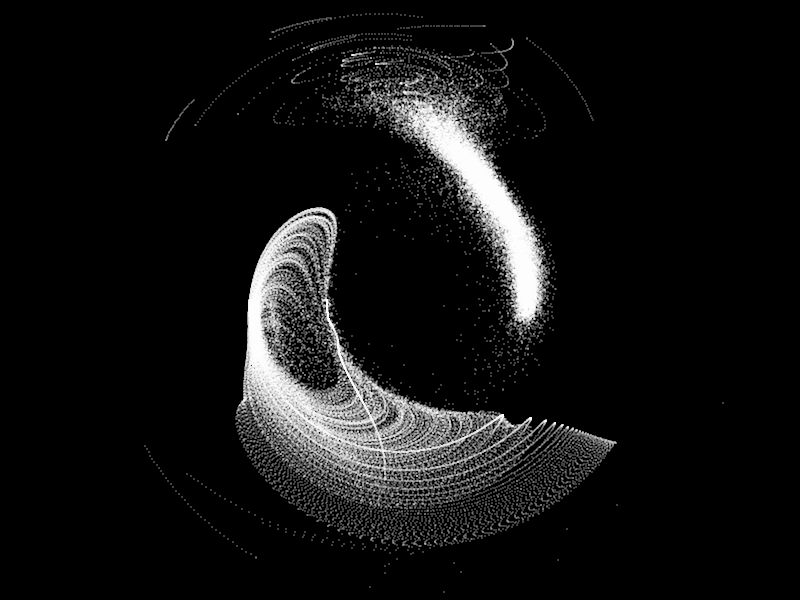FD [Dual Vortex]FD [Seashell]TD [Atom]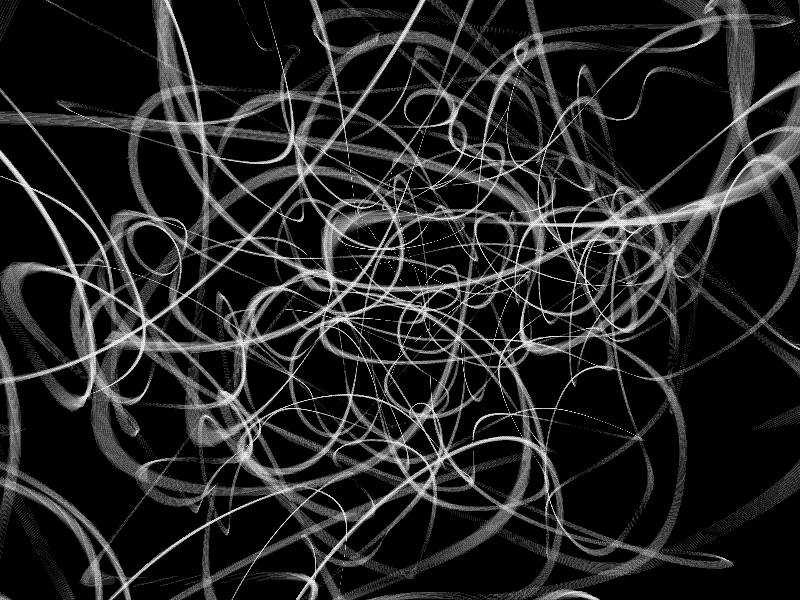TD [String Field]FD [Wormhole]